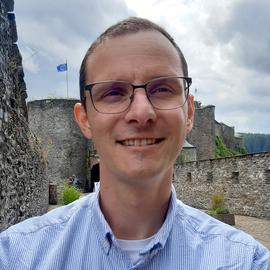# Rank-one projection models in optics: from lensless interferometry to optical sketching

Date
Sep 11, 2023 —
Location
ENS Lyon, France

Abstract: Many fields of science and technology face an ever-growing accumulation of “data”, such as signals, images, video, or biomedical data volumes. As a result, many techniques and algorithms, such as principal component analysis, clustering or random projection methods, have been devised to summarize these objects in reduced representations while preserving key information in this compression. In other contexts, it is the physics of the considered application that imposes us to indirectly observe an object of interest (such as biological cells, human brains or black holes) through such distorted representations while still being able to correctly image this object. In this presentation, we will review two representative applications of these two facets of data reduction models, namely interferometric lensless imaging (LI) with a multicore fiber (MCF), and quadratic random sketching with an optical processing unit (OPU). Their common feature lies in their mathematical models; they both rely on a specific data reduction called rank-one projection (ROP). Mathematically, a ROP model appears as soon as a structured data matrix—carrying information about a given object or data—is summarized by a series of joint left and right multiplication with a set of sketching vectors. A decade ago, several researchers showed that, under certain conditions, one can recover a structured matrix from ROP observations if their number exceeds the intrinsic complexity of this matrix. We will see the specific features of each of these two applications. In the case of MCF-LI, we will explain how the physics of wave propagation defines a two-step sensing model: the combination of an interferometric matrix—encoding the spectral content of the object image—and a ROP observation model (induced by the complex amplitudes of each MCF cores). Regarding the second application, we will first discover the working principle of an OPU and in particular its correspondence with quadratic random projection of a data stream, that is, a ROP model applied to a specific lifting of the data in a higher-dimensional domain. We will then show how to perform simple signal processing tasks (such as deducing local variations in an image) directly on the OPU sketches, with proof-of-concept experiments defined over naive data classification methods.

This is a joint work with Olivier Leblanc (UCLouvain, Belgium), Mathias Hofer (Institut Fresnel, France), Siddharth Sivankutty (U. Lille, France), and Hervé Rigneault (Institut Fresnel, France), and Rémi Delogne (UCLouvain, Belgium), Vincent Schellekens (imec, Belgium), and Laurent Daudet (LightOn, France).